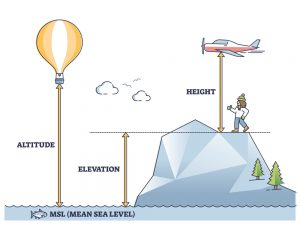# Altitude Vs. Elevation Vs. Height

You may be hiking and seeing the altitude reading on your altimeter device. But somehow, the term elevation pops up in your mind, and you realize that they are almost the same and do not know how to distinguish them. If you are wondering what actually altitude and elevation mean, then this page may help you a little to differentiate them.

## Simply Explained the Difference Between Elevation, Altitude, & Height

Actually, these terms are the vertical distance (height) between two points. Both of them start from the lowest point which is the mean sea level. But their highest points are different. And this is what makes them different. Altitude highest point is an object, while elevation is ground (area). Meanwhile, the different distance between the ground and flying object can be called as height.

In other words,

As said before, the term elevation is the distance of the area/ground from the mean sea level. This distance must be inline with gravitational line; no tilt. It has to start from this reference point (which is the mean sea level) and go upward to that ground.

In contrast, the term altitude is the distance of an object from the mean sea level. In this case, the object is usually above the ground or a flying object such as a plane, sky flyer, etc.Therefore, if you are sky flying, what your handheld altimeter measures is your altitude. It constantly changes as you fly. You start from a certain elevation and end at a certain elevation. In this case, your device should measure the elevation change as well (the difference in elevation from start to finish).

In comparison, height is the distance from any starting point to the final point. Unlike altitude and elevation that starts from mean sea level (as the reference point), height is no matter the starting point.

## Calculating Altitude if Elevation and Height are Known

Let us listen to the following little example of a boy in country A that travels to country B.

We have to know the elevation of country A is above the main sea level. So, you could search the data and find it 507 meters above the main sea level. Country A’s elevation is 507 meters.

The boy travels y plane. The current plane height is 10,000 meters. Measure that boy/plane altitude at that current time?

As known before, the elevation is 507 meters. So, the altitude is the elevation plus the plane’s height from the ground. The altitude will be equal to 10507 meters (507 m + 10000 m)

Altitude = elevation + height of the object

## Negative Altitude Vs. High Altitude

Altitude can be negative in some cases depending on where the object is? It may be above sea level or below sea level. For example, if a bird is diving into the sea, the altitude will become negative. That is negative because the bird is now below sea level. The higher altitude is when the height above the main sea level is 2400 meters or 8000 feet.

## Instruments to Measure Altitude

To measure the altitude of an area, you can use an altimeter.

There are a variety of altimeter models you can choose from. Most of them measure the altitudes by measuring the air pressure. As the air pressure decreases by about 1 millibar per 10 meters of height increasing from the mean sea level, this device is pretty well to serve you with altitude information.

An altimeter is a very important piece of equipment for the navigation of aircraft. It is commonly located on the dashboard of a craft. Altimeter watch is also available to buy. Some mechanical altimeters can be purchased and installed on your car dashboard.

## Comparison Chart of the Differences Between Altitude, Elevation, and Height

 Altitude Elevation Height 1.      How high an object is above the main sea level. 1.      Height of the place or an area above the main sea level. 1.      Measurement of an object from the reference point to the final point. 2.      To calculate altitude Altitude= elevation + height of an object above the ground. 2.   To calculate elevation Elevation= height of an area above the main sea level. 2.   To calculate distance Height= add an initial point to the final point distance. 3.      Altitude can be negative Like under seawater. 3.   Elevation can be negative because some deserts are below sea level; therefore, elevation is negative in these places. 3.    In physics, height can be negative in the basement of a building its height is negative. 4.      The Instrument used to measure the altitude is an altimeter. 4. The Instrument used to measure the elevation or absolute altitude is GPS. 4. Instrument used to measure the height of an object is an altimeter. 5.      Atmospheric pressure decreases as altitude increases. 5. Atmospheric pressure decreases as elevation increases. 5. Atmospheric pressure decreases as height increases. 6.      Unit measurement = meter. 6.    Unit measurement = meter. 6.    Unit measurement = meter.